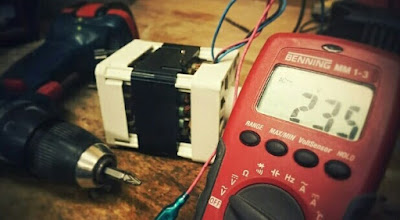### Ticker

6/recent/ticker-posts

# Instruments and Measurements of Units, Dimensions and Standards

## UNITS, DIMENSIONS AND STANDARDS

### Nature of units :-

In order to record or to compare magnitude of quantities, some one magnitude of each kind must be taken as basis or unit.

The unit may also be defined as the standard measure of each kind of of physical quantity.Electrical Instruments

Each unit, eighter must be Represented by a physical Standard of some kind, natural or artificial or must be derived from a combination of other units Represented by such Standards.

### Fundamental and Derived Quantities :-

Experience show that three basic idea sufficient to describe or units are sufficient to describe quantitatively all the phenomena encountered in Mechanical Science.

These are length, mass and time and are called fundamental quantities.

All other quantities are expressed in terms of the fundamental Quantities and are, therefore, called the Derived Quantities.

Velocity, force acceleration, power, energy etc., Are all Derived quantities.

### Dimensions :-

Each quantity has a quality which "distinguishes". It from the other. This unique quality is called "dimensions".

A derived unit is recognized by the dimensions and can be defined as the complete algebraic formula for the Derived unit.

The various powers of the fundamental units Represent the dimensions of any Derived unit.

The expression indicating the nature of Derived quantities in terms of fundamental
[L], [M] and [T] are known as dimensional Equations and are helpful in

1) Conversion from one System of units to another one

2) Derivation of Equations for physical quantities and

3) checking the accuracy of an Equation.

### SI System of Units :-

This is the latest from of metric system and absorbs in it the RMKSA System.

The SI System is, in fact, simply the RMKSA System Expanded by adding the degree

a) Kelvin

b) Candela

c) Mole as basic units of temperature

d) Luminous Intensity

e) Amount of Substances respectively.

SI System of units has two supplementary units viz.

( for  solid angle ) in addition to seven base Units of

1) Meters

2) Kg

3) Second

4) Amperes,

5) Kelvin

6) Candela and mole for length

7) Mass, time and Current

8) Thermodynamic Temperature

9) Luminous Intensity and amount Substances respectively.

The SI System is a Comprehensive, logical and coherent system designed for use in all branches of science, Engineering and Technological.

### Absolute Measurement of Electrical Quantities :-

Measurements of Electrical Quantities in terms of the units of Mass, length and time
Are called absolute Measurement.

These are necessary only for the purpose of establishment, or varification of the reference Standards.